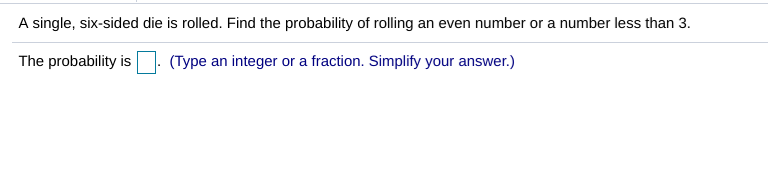# A single, six-sided die is rolled. Find the probability of rolling an even number or a number less than 3.The probability is(Type an integer or a fraction. Simplify your answer.)

Question
197 viewshelp_outlineImage TranscriptioncloseA single, six-sided die is rolled. Find the probability of rolling an even number or a number less than 3. The probability is(Type an integer or a fraction. Simplify your answer.) fullscreen
check_circle

Step 1

Addition rule of probability indicates the happening either of the events that are involved.

Consider two events A and B, the happening of either of the events A or B is obtained as follows:

Step 2

Obtain the probability of rolling an even number or a number less than 3.

A six sided die will have the numbers 1, 2, 3, 4, 5 and 6.

The outcomes of the sample space are S = {1, 2, 3, 4, 5, 6}.

That is, the total number of possible outcomes is n(S) = 6.

Denote the event “even number” as A.

The possible outcomes for the event “even number” are {2, 4, 6}.

That is, the number of favourable outcomes for A is n (A) = 3.

Denote the event “number less than 3” as B.

The possible outcomes for the...

### Want to see the full answer?

See Solution

#### Want to see this answer and more?

Solutions are written by subject experts who are available 24/7. Questions are typically answered within 1 hour.*

See Solution
*Response times may vary by subject and question.
Tagged in

### Other